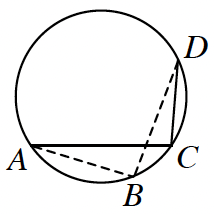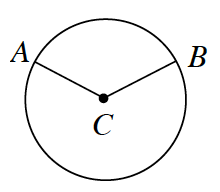### Home > GC > Chapter 10 > Lesson 10.2.2 > Problem10-70

10-70.

Review what you know about the angles and arcs of circles below.

1. A circle is divided into nine congruent sectors. What is the measure of each central angle?

What is the total angle measure of a circle?

2. In the diagram at right, find $m\overarc{AD}$ and $m∠C$ if $m∠B=97°$.

How are inscribed angles related to intercepted arcs?

$m\overarc{AED}=2\left(97°\right)=194°$$m\overarc{AD}=2\left(97°\right)=194°$

$m\angle C=0.5\left(194°\right)=97°$3. In $⊙C$ at right, $m∠ACB=125°$ and $r = 8$ inches. Find $m\overarc{ A B }$ and the length of $\overarc{ A B }$. Then find the area of the smaller sector.

How does the angle measure relate to the arc measure?

Intercepted arcs are of equal measure to their corresponding central angles.

$m\overparen{AB}=m\angle {ACB}=125°$

Circumference of the Whole Circle $= 2πr = 2π\left(8\right) = 16π \; \text{inches}$

Area of Circle $= πr^2 = π(8)^2 = 64π \; \text{in}^2$

$m\overarc{AB}=\frac{125°}{360°}\cdot16\pi=\frac{2000}{360}\pi\approx17.5\text{ in}$

Area of Sector $=\frac{125°}{360°}\cdot64\pi=\frac{8000}{360}\pi\approx69.8\text{ in}^2$